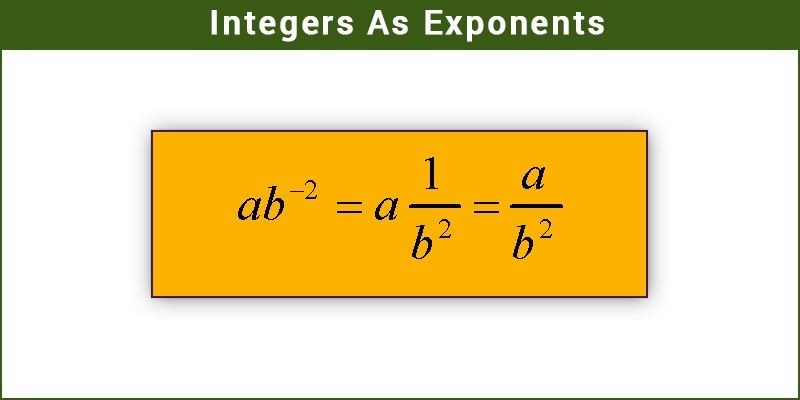Checkout JEE MAINS 2022 Question Paper Analysis : Checkout JEE MAINS 2022 Question Paper Analysis :Exponents are used to showing repeated multiplication of a number by itself. Writing large numbers sometimes becomes tedious. In large mathematical expressions, they occupy more space and take more time. This issue is resolved by the use of exponents. For example, 7 × 7 × 7 can be represented as

$$\begin{array}{l} 7^3 \end{array}$$
. In this example, the exponent is ‘3’ which stands for the number of times the value is multiplied by itself. The number 7 is called the base which is the actual number that is getting multiplied. For instance, the speed of light is 300000000 m/s. This can be simply written as 3 ×
$$\begin{array}{l} 10^8 \end{array}$$
m/s (approximate value). This process of using exponents is called as ‘raising to a power’ where the exponent is the power. In this article, we are going to discuss the difference between exponents and powers, and the detailed explanation about the integers as exponents with important rules and examples.

## What is Meant by Integers as Exponents?

In Mathematic, the integers exponents are the exponents that should be an integer. It can be either a positive integer or a negative integer. In this, the positive integer exponents describe how many times the base number should be multiplied by itself. Whereas the negative integer exponents first describe flipping the numerator and the denominator value and define to multiply the number by itself for the number of times mentioned there.

## Exponents Vs Powers

We know that the expression 6 x 6 can be calculated, but the expression can also be written in a short manner that is known as exponents.

6.6 = 6^2

The expression that describes repetitive multiplication of same value is known as power. The value 6 is known as base or power and the number 2 is known as an exponent. It corresponds to the number of times the base is operated as a factor.

## Integer Exponent Rules

 Rule name Rule Example Product rule a n· am = an+m 23 · 24 = 23+4 = 128 a n · b n = (a · b) n 32 · 42= (3·4)2 = 144 Quotient rule a n/ a m = an-m 25 / 23 = 25-3 = 4 a n / b n = (a / b)n 43 / 23= (4/2)3 = 8 Power rule (bn)m = bnm (23)2 = 23.2 = 64 m√(bn) = bn/m 2√(26) = 26/2 = 8 b1/n = n√b 81/3  = 3√8 = 2 Negative exponent b-n= 1 / bn 2-3 = 1/23 = 0.125 Zero rule b0 = 1 60 = 1 0n = 0 , for n>0 06= 0 One rule b1 = b 71 = 7 1n = 1, n= even number 18 = 1 Minus one rule (-1)n= -1 , n= odd number (-1)5 = -1 Derivative rule (xn)’ = n·x n-1 (x3)’ = 3·x3-1 Integral rule ∫ xndx = xn+1/(n+1)+C ∫ x2dx = x2+1/(2+1)+C

### Integers with Positive and Negative Exponents

We know that

$$\begin{array}{l} 20^2 \end{array}$$
= 20 × 20 = 400

=>

$$\begin{array}{l} 20^1 \end{array}$$
=
$$\begin{array}{l} \frac {400}{20}\end{array}$$
= 20

=>

$$\begin{array}{l} 20^0 \end{array}$$
=
$$\begin{array}{l} \frac {20}{20}\end{array}$$
= 1

So,

$$\begin{array}{l} 20^{-1} \end{array}$$
=
$$\begin{array}{l} \frac {1}{20}\end{array}$$

Similarly,

$$\begin{array}{l} 20^{-2} \end{array}$$
=
$$\begin{array}{l} \frac {1}{20}\end{array}$$
÷ 20 =
$$\begin{array}{l} \frac {1}{20}\end{array}$$
×
$$\begin{array}{l} \frac {1}{20}\end{array}$$
=
$$\begin{array}{l} \frac {1}{20^2}\end{array}$$

$$\begin{array}{l} 20^{-3} = \frac {1}{20^3}\end{array}$$

In general we can say that for any non-zero integer say ‘a’ ,

$$\begin{array}{l} a^{-3} = \frac{1}{a^m} \end{array}$$
, where m is the positive integer.
$$\begin{array}{l} a^{- m} \end{array}$$
is also the multiplicative inverse of
$$\begin{array}{l} a^m\end{array}$$
.

### Integers as Exponents Examples

Example 1:

Find the multiplicative inverse of

$$\begin{array}{l} 9^{-4} \end{array}$$

Solution:

$$\begin{array}{l} 9^{-4} \end{array}$$
=
$$\begin{array}{l} \frac {1}{9^4} \end{array}$$

Therefore the multiplicative inverse of

$$\begin{array}{l} \frac {1}{9^4} \end{array}$$
is
$$\begin{array}{l} 9^{4} \end{array}$$
.

Example 2:

Find the multiplicative inverse of

$$\begin{array}{l} 7^{2} \end{array}$$
.

Solution:

$$\begin{array}{l} 7^{2} \end{array}$$
=
$$\begin{array}{l} \frac {1}{7^{-2}} \end{array}$$

The multiplicative inverse of

$$\begin{array}{l} 7^{2} \end{array}$$
is
$$\begin{array}{l} 7^{-2} \end{array}$$
.

Example 3:

Expand the number 12345 in the exponent form.

Solution:

The number 12345 can be expressed as:

12345 = 1 × 10000 + 2 × 1000 + 3 × 100 + 4 × 10 + 5 × 1

=> 12345 = 1 ×

$$\begin{array}{l}10^4 ~+~ 2 ~×~ 10^3~ +~ 3~ ×~ 10^2 ~+~4~ ×~ 10^1~ +~ 5~ ×~ 10^0\end{array}$$
(any number raised to the power 0 is equal to 1).

Similarly, this method can be employed to decimal numbers also.

Example 4:

Expand the number 987.65 in the exponent form.

Solution:

The number 987.65 can be represented as:

$$\begin{array}{l}9~ ×~ 10^2~ +~ 8~ ×~ 10^1~ +~ 7~ ×~ 10^0~ +~ 6~ ×~ 10^{-1}~ +~ 5~ ×~ 10^{-2}\end{array}$$